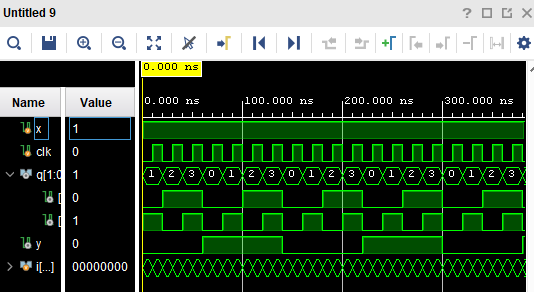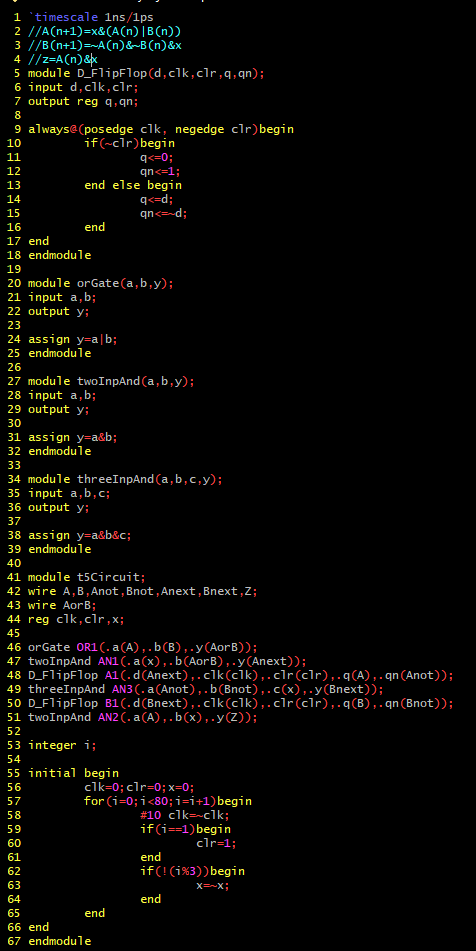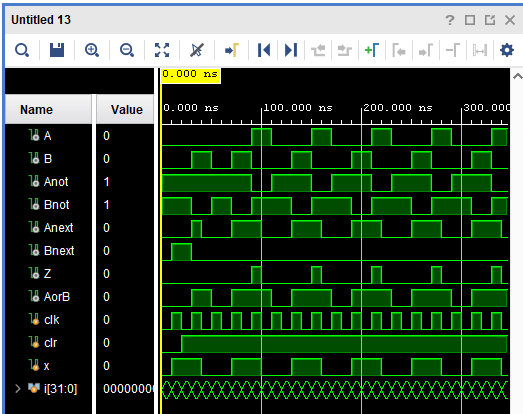CE433 Embedded Devices Spring 2022
Homework 5 - Sequential Circuits
Name: Ryan Jeanes

Email: rejeanes@fortlewis.edu

Homework 5 - Sequential Circuits
Introduction
This assignment focused  on building logical equations from sequential circuitry and vice versa.
Results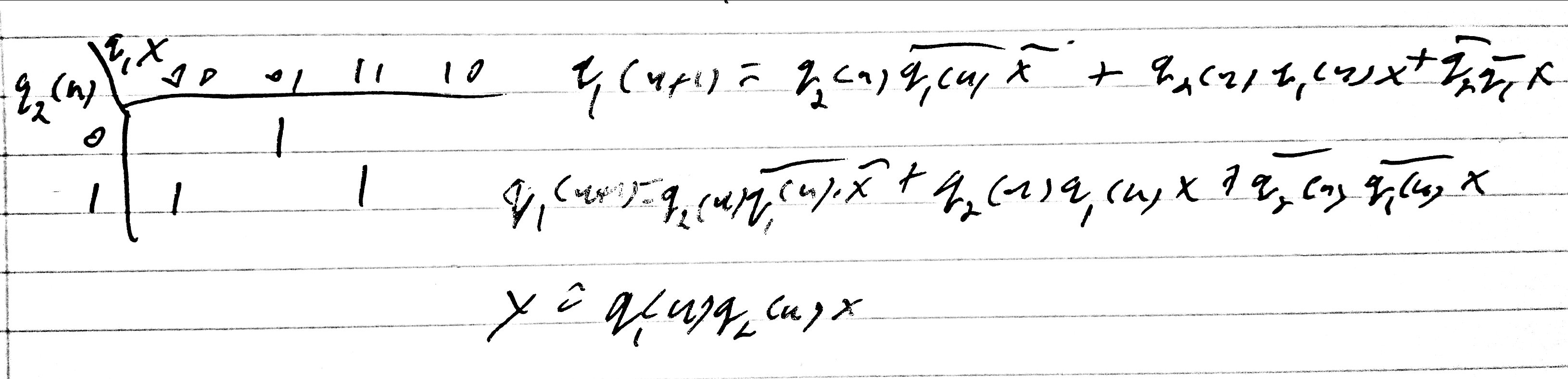Figure 1 - Equations for state table given in section 1, with q1(n+1) derived from k-map.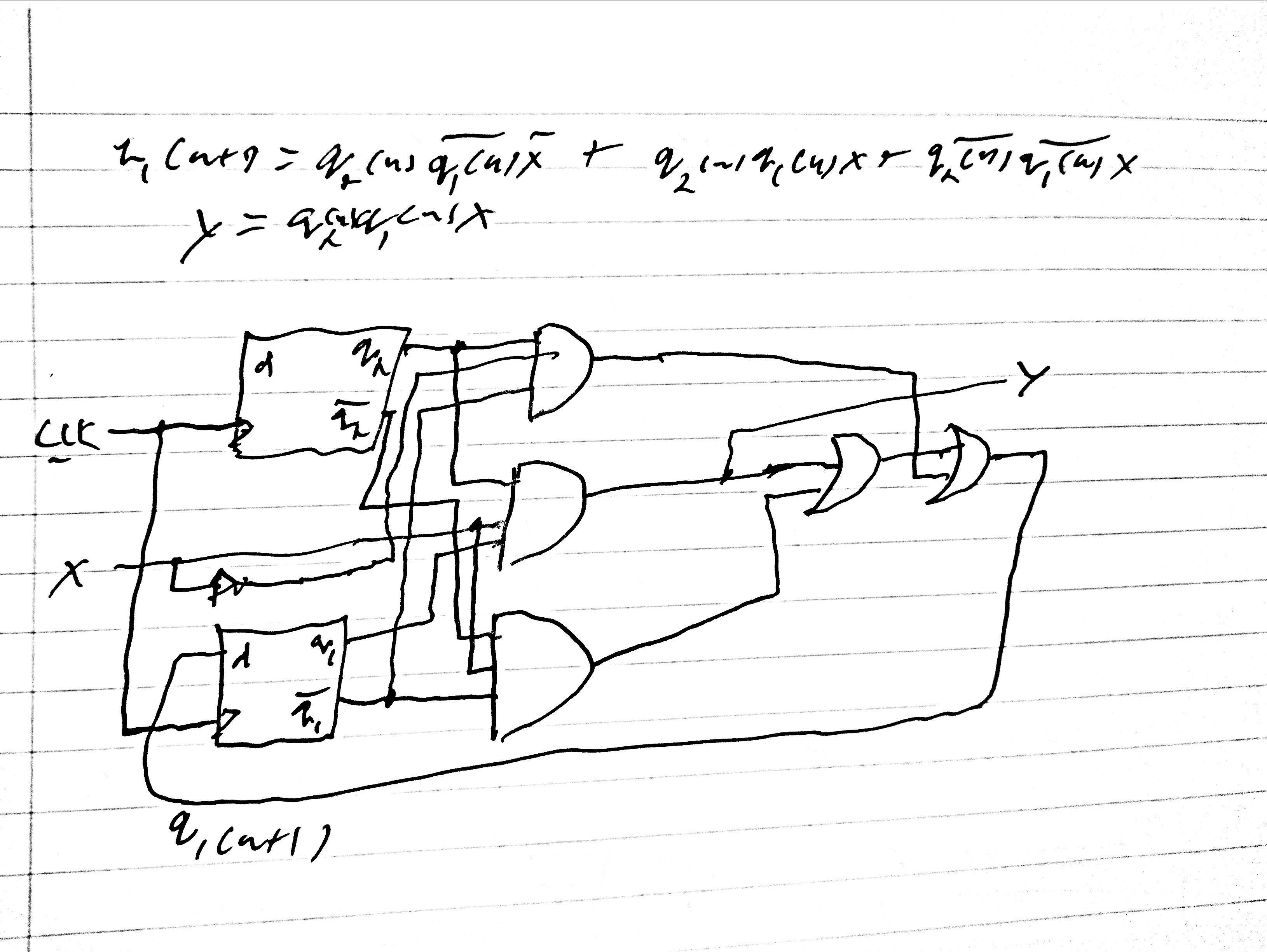Figure 2 - Drawn circuit derived from the equations.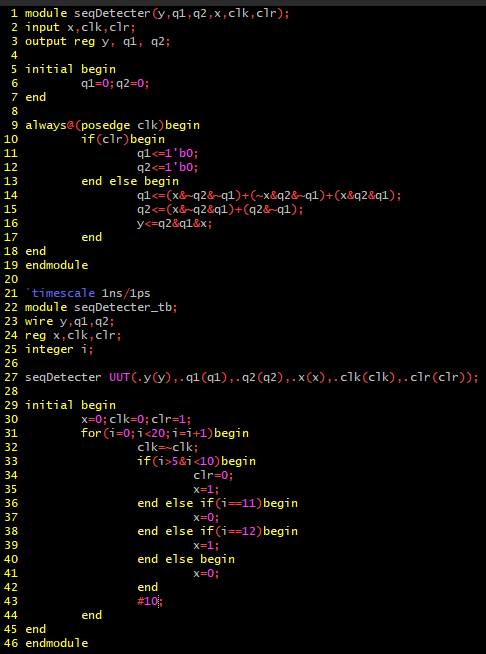Figure 3 - Code for sequential detecter shown in section 3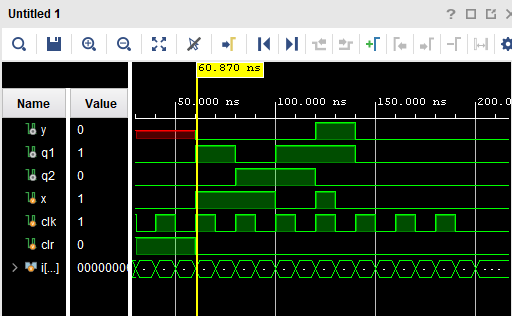Figure 4 - Simulation for sequential detecter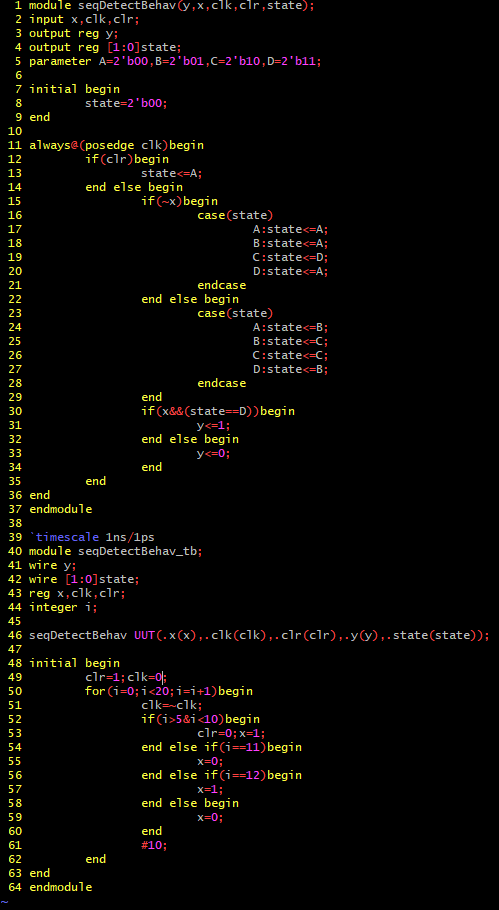Figure 5 - Code for sequential detecter using behavioral modeling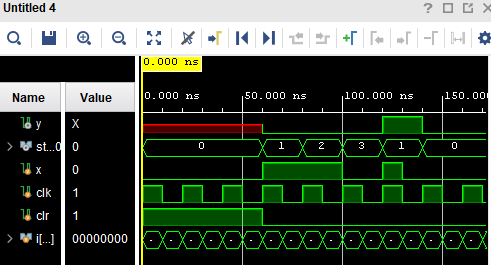Figure 6 - Simulation for sequential detecter using behavioral modeling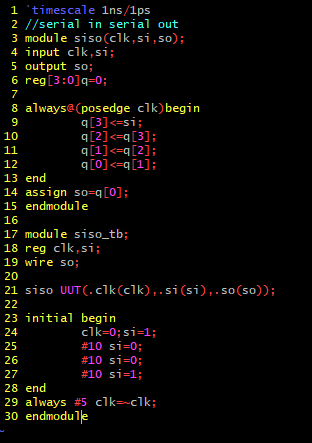Figure 7 - Code for SISO register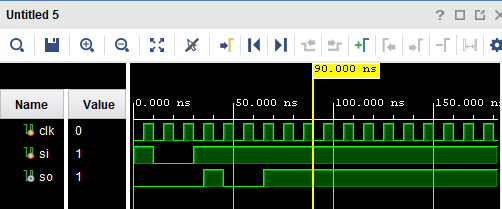Figure 8 - Simulation for SISO register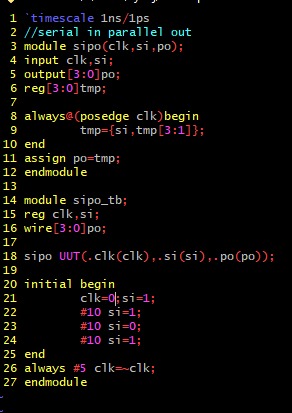Figure 9 - Code for SIPO register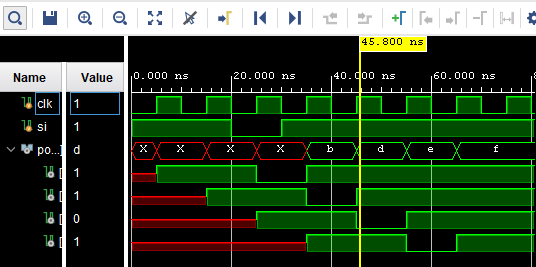Figure 10 - Simulation for SIPO register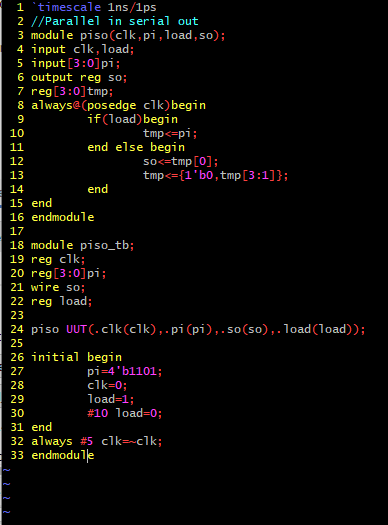Figure 11 - Code for PISO register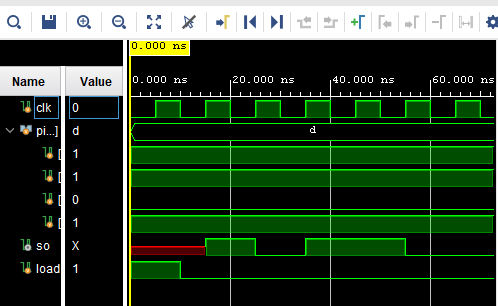Figure 12 - Simulation for PISO register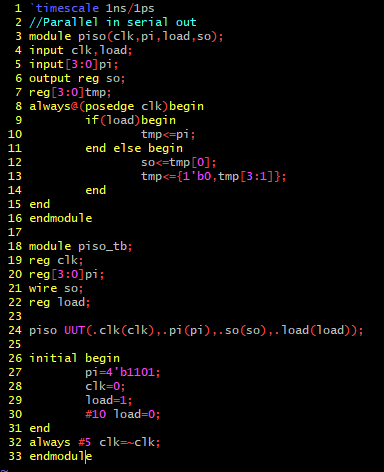Figure 13 - Code for PIPO registerFigure 14 - Simulation for PIPO register